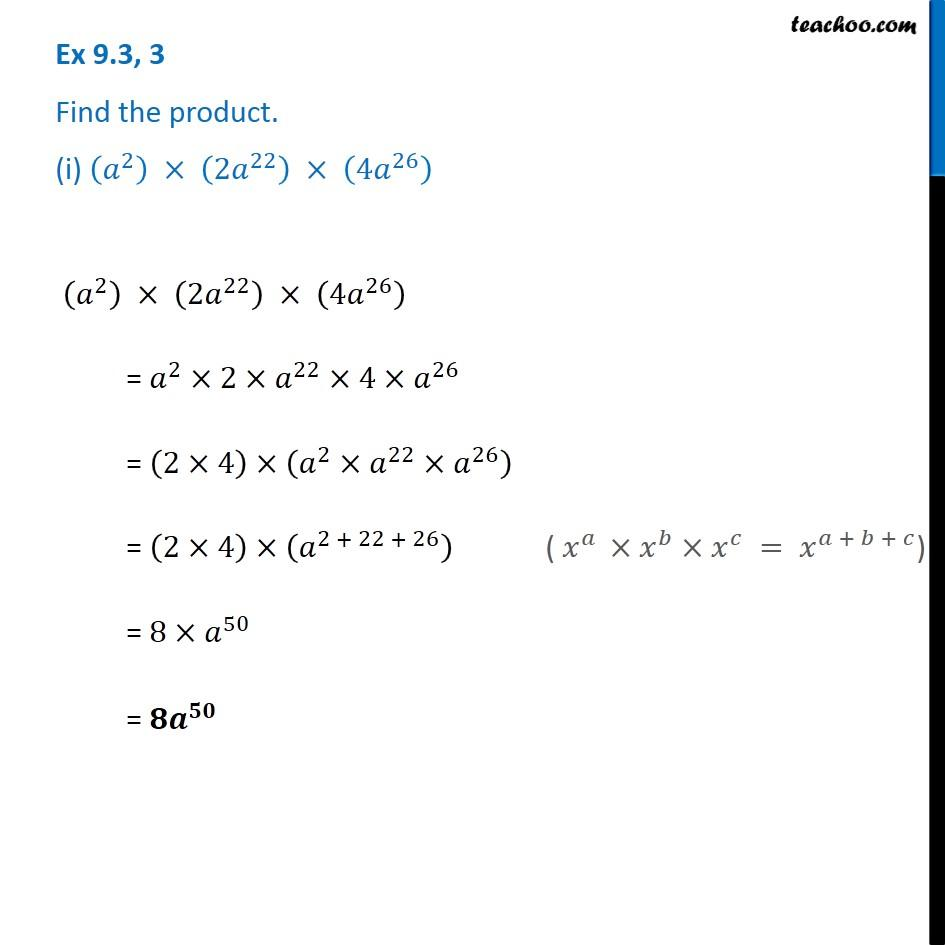Multiplication of Monomials by Binomials and Trinomials

Chapter 8 Class 8 Algebraic Expressions and Identities
Concept wiseLearn in your speed, with individual attention - Teachoo Maths 1-on-1 Class

### Transcript

Ex 8.3, 3 Find the product. (i) (𝑎^2 ) × (2𝑎^22 ) × (4𝑎^26 ) (𝑎^2 ) × (2𝑎^22 ) × (4𝑎^26 ) = 𝑎^2×2×𝑎^22×4×𝑎^26 = (2×4)×(𝑎^2×𝑎^22×𝑎^26 ) = (2×4)×(𝑎^(2 + 22 + 26) ) ( 𝑥^𝑎 ×𝑥^𝑏×𝑥^𝑐 = 𝑥^(𝑎 + 𝑏 + 𝑐)) = 8×𝑎^50 = 𝟖𝒂^𝟓𝟎